## Example Questions

### Example Question #1 : How To Find The Radius Of A Sphere

A cube with volume 27 cubic inches is inscribed inside a sphere such that each vertex of the cube touches the sphere. What is the radius, in inches, of the sphere?

Possible Answers:

9

8.5

(3√3)/2 (approximately 2.60)

√3/2 (approximately 1.73)

Correct answer:

(3√3)/2 (approximately 2.60)

Explanation:

We know that the cube has a volume of 27 cubic inches, so each side of the cube must be ∛27=3 inches. Since the cube is inscribed inside the sphere, the diameter of the sphere is the diagonal length of the cube, so the radius of the sphere is half of the diagonal length of the cube. To find the diagonal length of the cube, we use the distance formula d=√(32+32+32 )=√(3*32 )=3√3, and then divide the result by 2 to find the radius of the sphere, (3√3)/2.

### Example Question #2 : How To Find The Radius Of A Sphere

The surface area of a sphere is 100π square feet.  What is the radius in feet?

Possible Answers:

25

5

π

100

10

Correct answer:

5

Explanation:

S = 4π(r2)

100π = 4π(r2)

100 = 4r2

25 = r2

5 = r

### Example Question #3 : How To Find The Radius Of A Sphere

What is the radius of a sphere with a surface area of 16?

Possible Answers: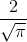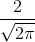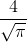Correct answer:Explanation:

In order to find the radius, first write the surface area formula for a sphere and substitute the surface area.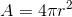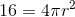Divide byon both sides in order to isolate the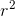term.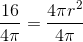Simplify both sides of the equation.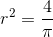Square root both sides.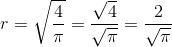The radius is.

### All SAT Math Resources